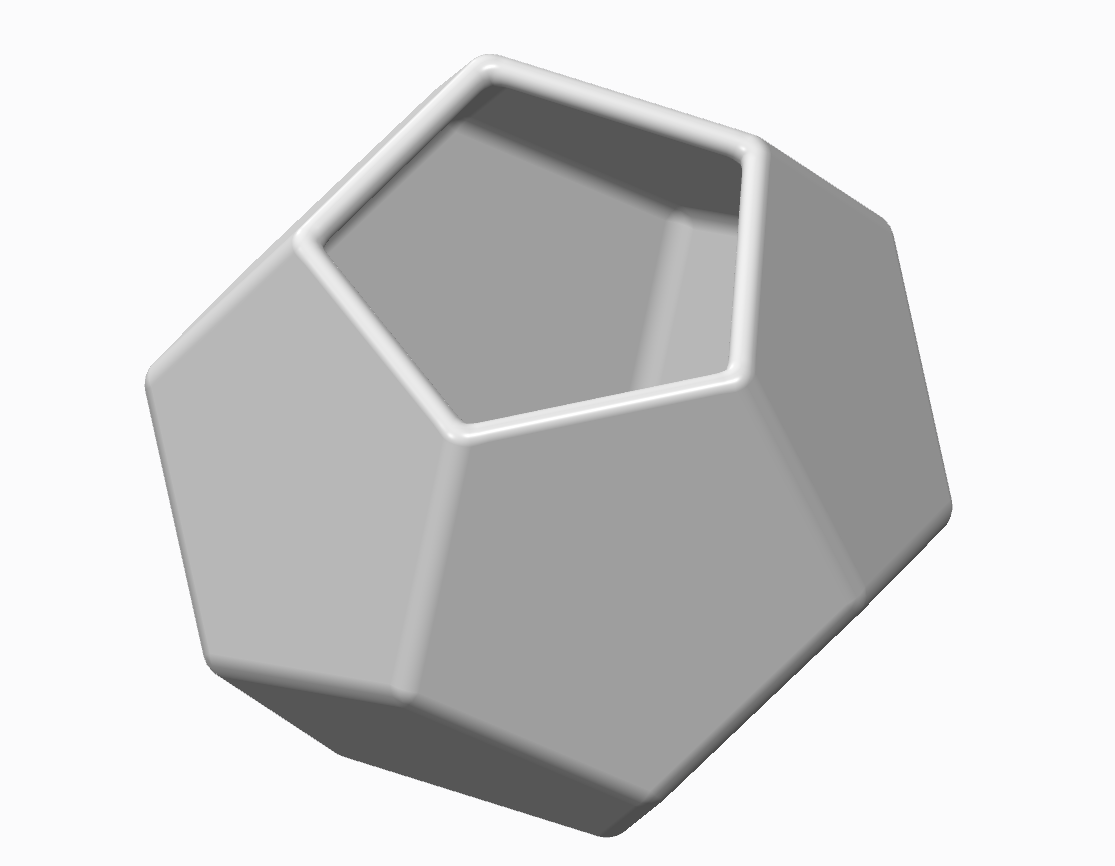# Tutorial - How to make dodecahedron pot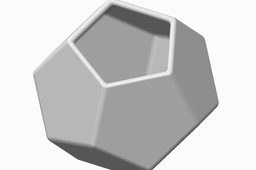That's what I did.

1. ### Step 1:

phi=2*atan((1+sqrt(5))/2)

a=100

Draw pentagon, where a is a radius of circumscribed circle.

Create auxiliary datum plane passing trough the rib at an angle equal to the parameter phi.

Draw second pentagon.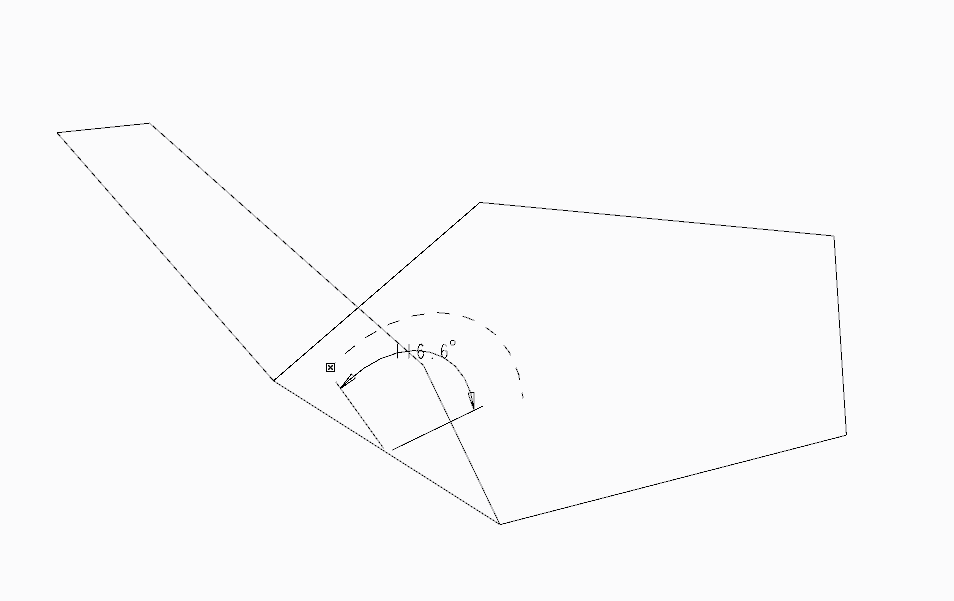2. ### Step 2:

Make surfaces of them.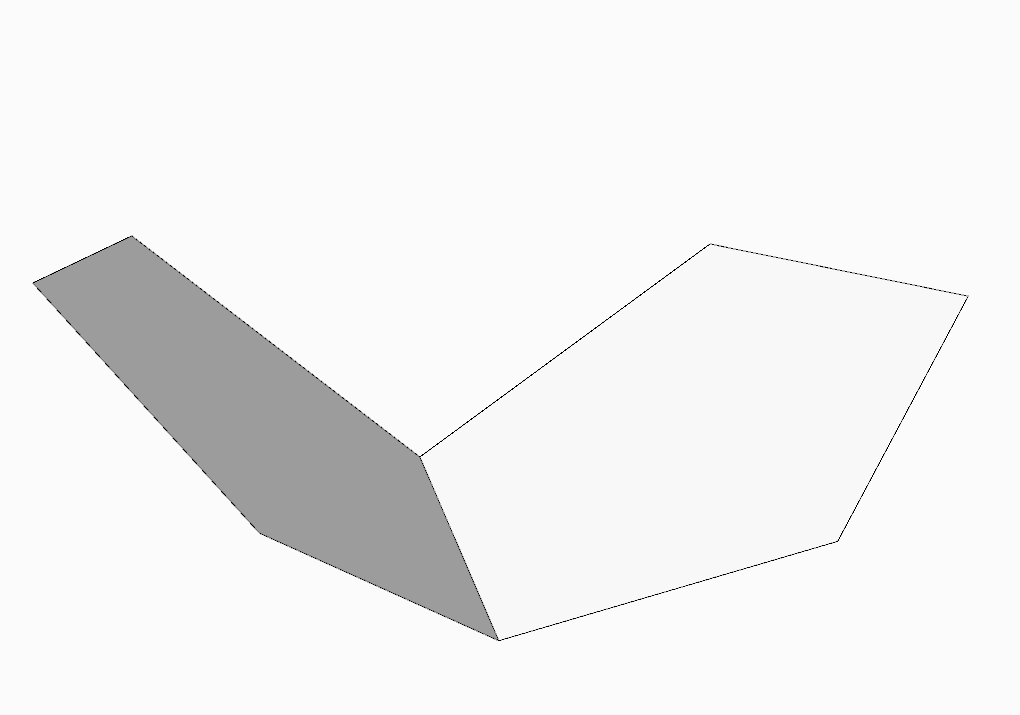3. ### Step 3:

Do array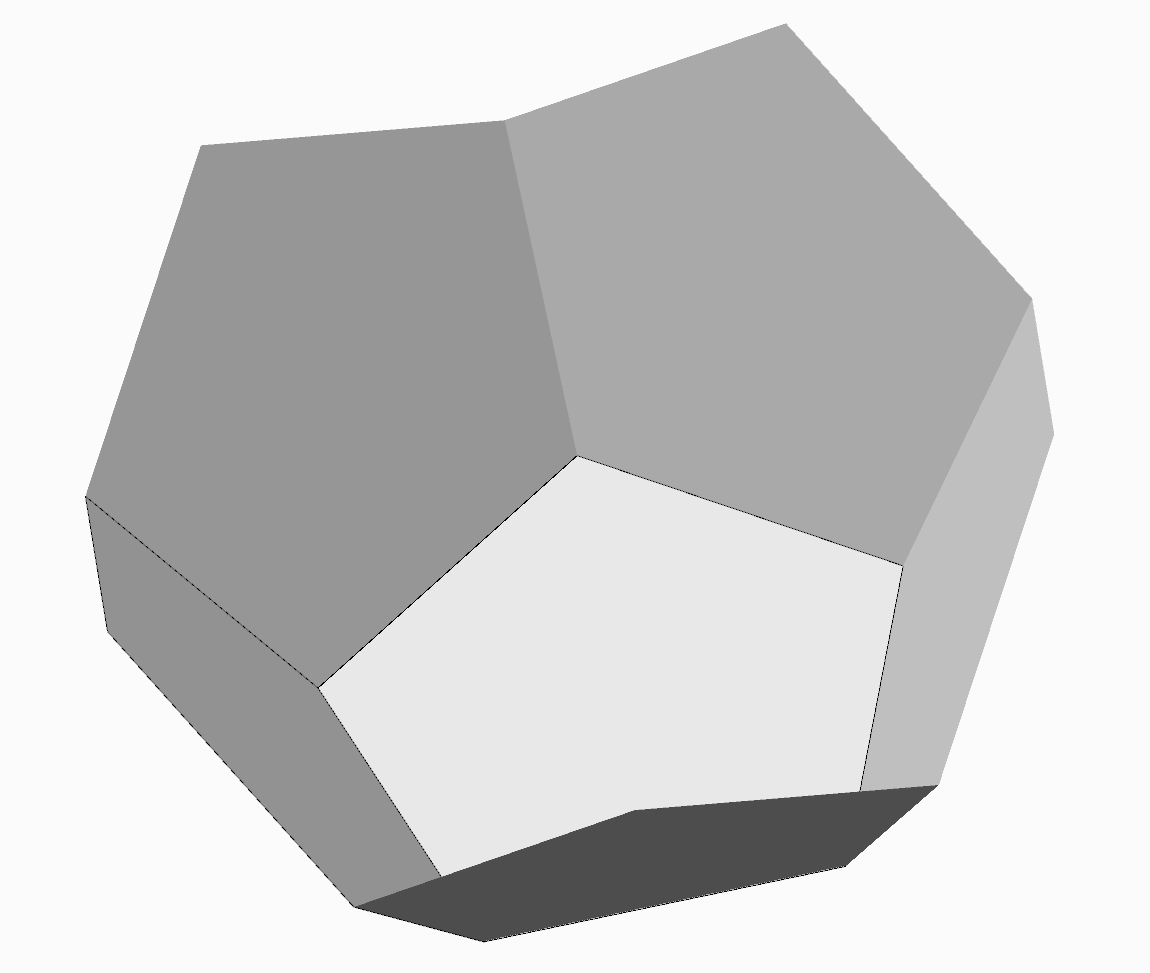4. ### Step 4:

Do copy five surfaces with translation by the amount equal to parameter (2*rad) along Y-axis. The axis Y - perpendicular to the plane of the first pentagon. And rotation axis Z and X on180° and 36°, respectively.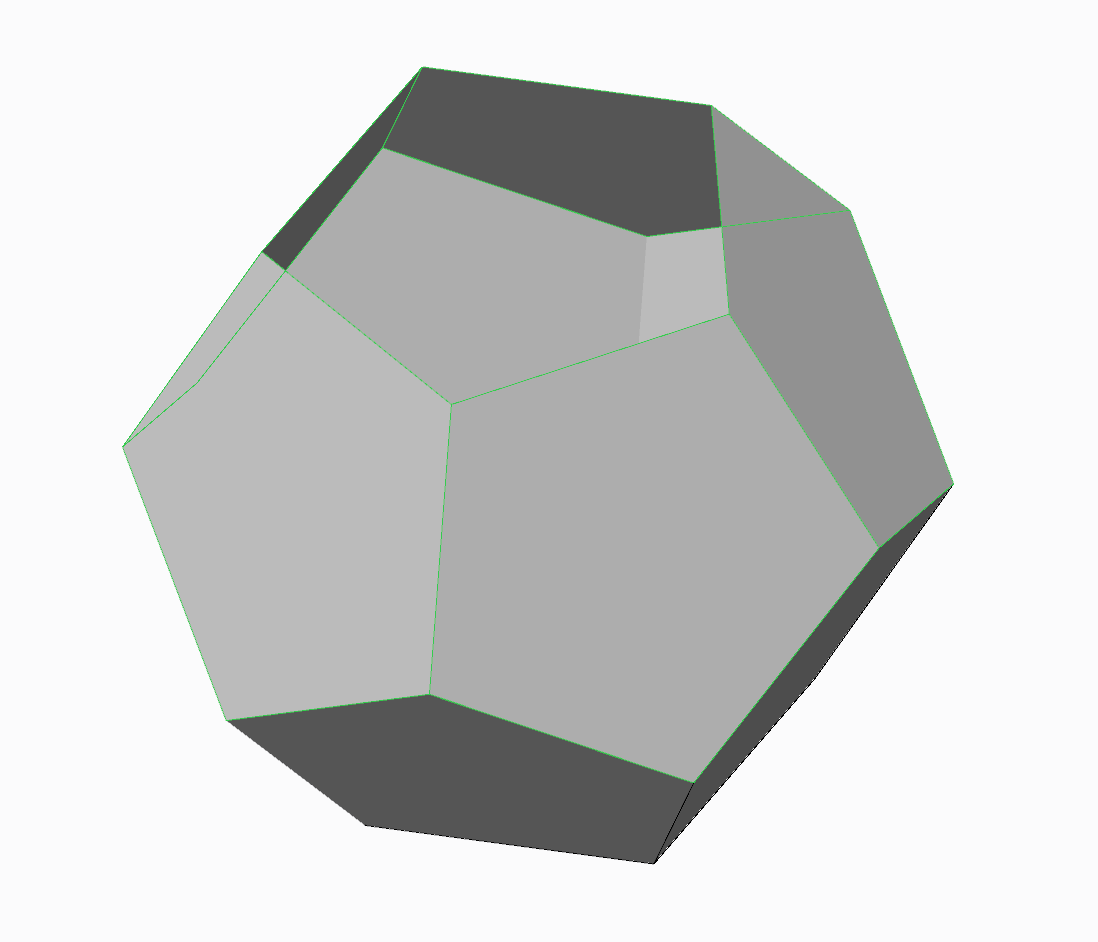5. ### Step 5:

Combine surfaces and do displacement inside.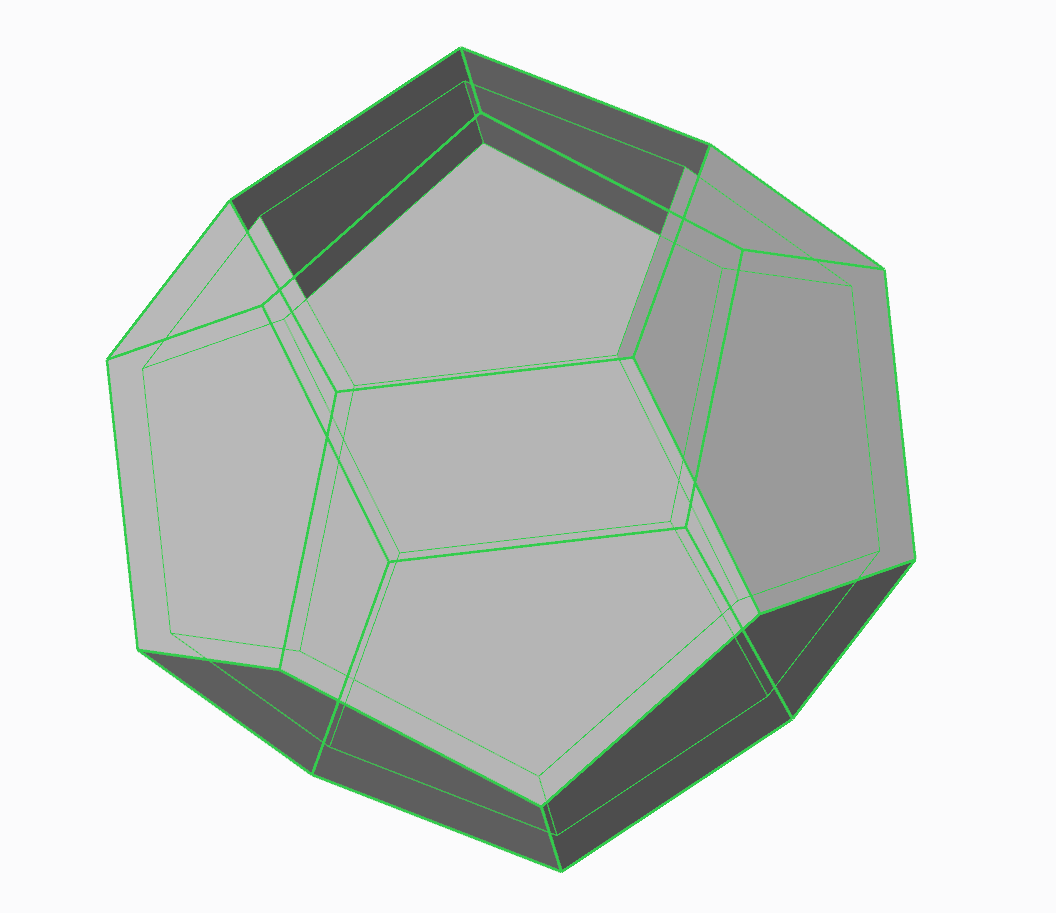6. ### Step 6:

Closing volume with surfaces. Make solid detail.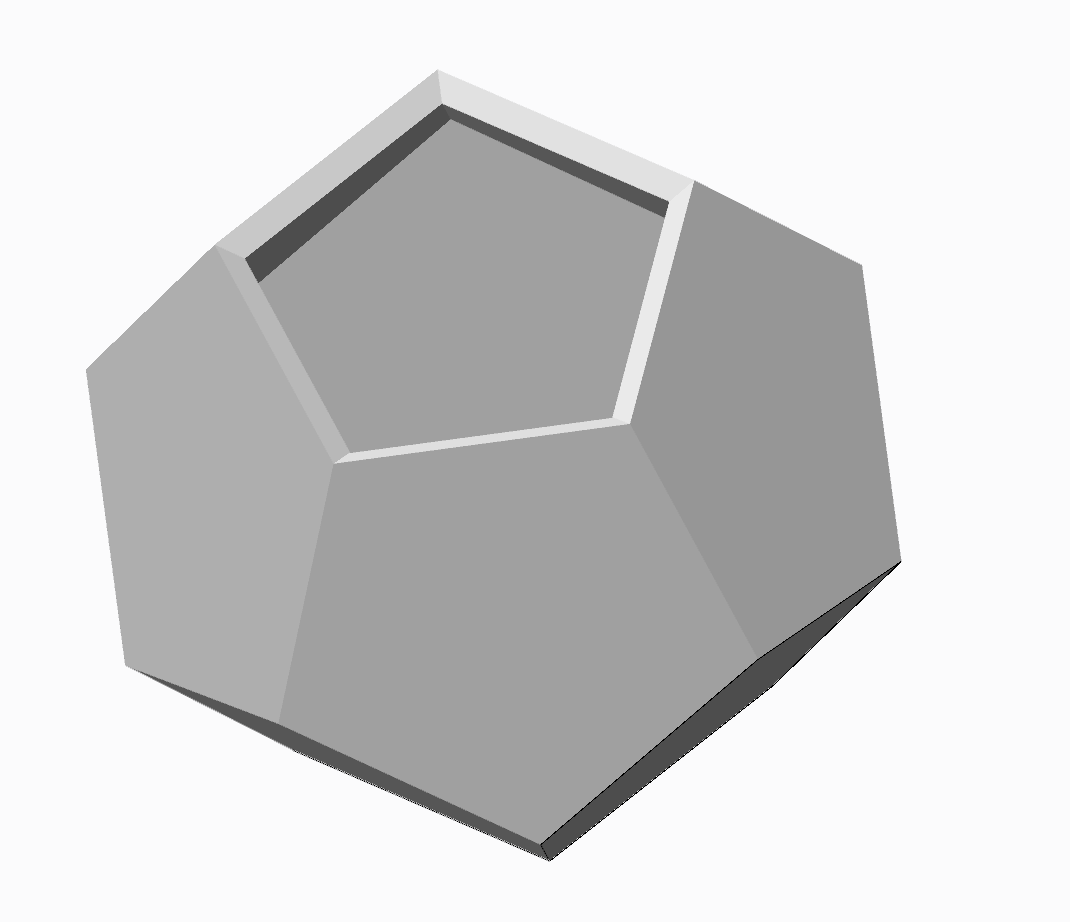7. ### Step 7:

Round, round and round.